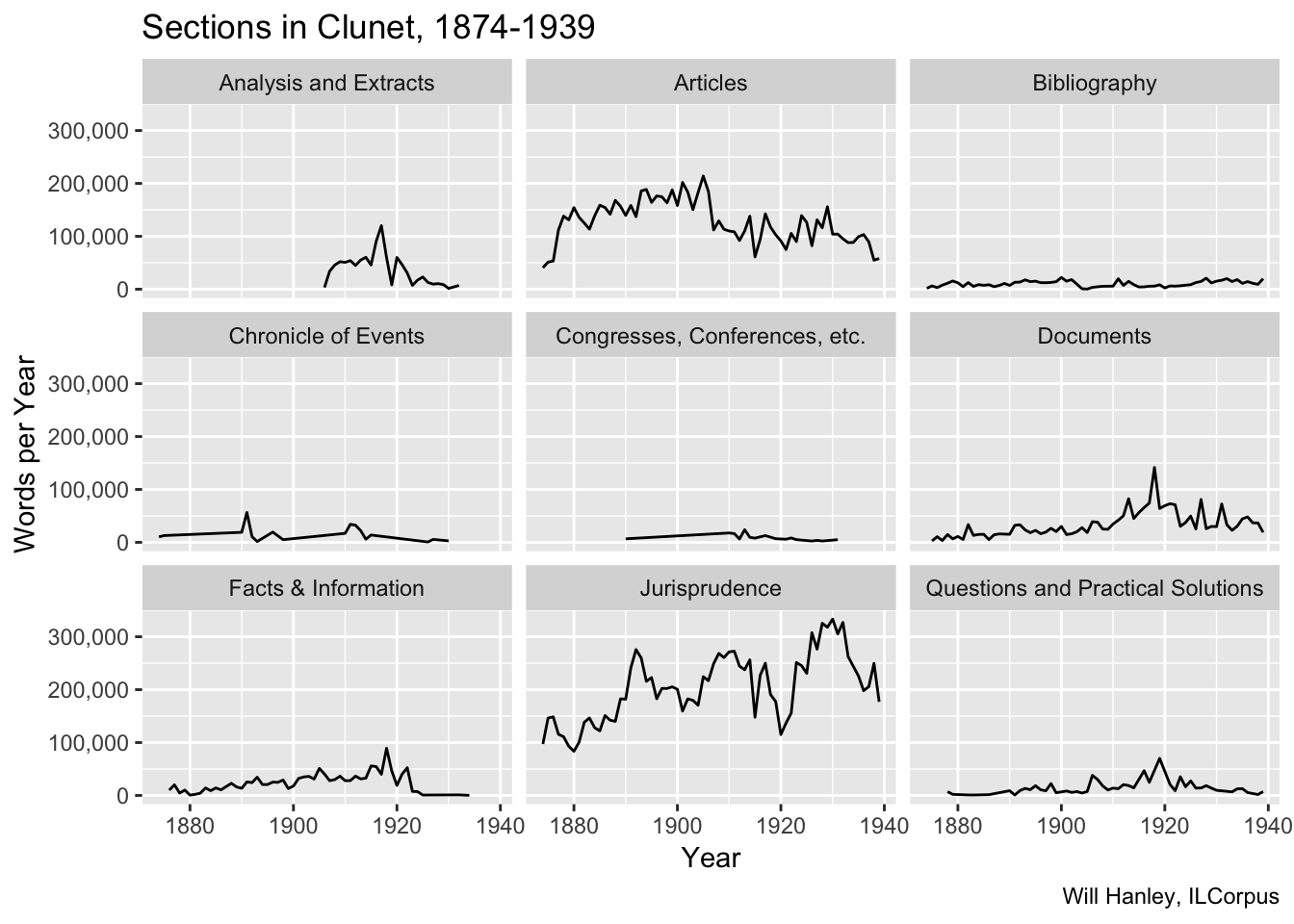# International Law Corpus

## Visualizations of international law periodical content, 1869-1939.

Clunet is subdivided into sections, each featuring a different genre of writing. If the text is mined without distinguishing between the different characters of these sections, results will be soupy. I have divided the text into these sections, following the journal’s own classification scheme. This table omits three sections that appeared for only a few years each: “Actualités”, “Revue des Revues”, and “Variétés”.Wartime spikes are particularly notable. Unlike other journals, Clunet increased publication during wartime.

Here’s a more impressionistic streamgraph of the same content (also including the minor divisons).

## Underlying code

``````library(xml2)
library(tidyverse)
library(tokenizers)
library(socviz)

# word count method - top level divs only
extract_data <- function(x){
#extract divs, get type attribute and
divs <- file %>% xml_find_all(".//d1:body/d1:div")
type <- divs %>% xml_attr("type")
feature <- divs %>% xml_attr("feature")
filename <- x %>% gsub(".+/GitHub/ilcorpus/journals/[a-z]+/[a-z,-]+/", "", .) %>% gsub(".xml", "", .)
year <- x %>% gsub(".+/GitHub/ilcorpus/journals/[a-z]+/[a-z,-]+/[a-z]+-", "", .) %>% gsub("-..xml", "", .)

#find the head and p for each div
#returns a list of data frames
output <- lapply(divs, function(d){
xml_text() %>%
paste(collapse=" ") %>%
gsub("\\s+", " ", .)
text <- d %>%
xml_find_all(".//d1:p") %>%
xml_text() %>%
paste(collapse=" ") %>%
gsub("\\s+", " ", .)
count <- length(tokenize_words(text)[])
})
#bind everything up into a tibble.
test_tibble <- cbind(tibble(filename = filename, year = as.numeric(year), seq = 1:nrow(answer), type = type, feature = feature), answer)
}

clunet_files <- dir("~/GitHub/ilcorpus/journals/clunet/clunet-issues", full.names = TRUE)

# run function and make tibble
clunet_divs <- lapply(clunet_files, extract_data)
one_list <- do.call(rbind,clunet_divs)
clunet_divs_tibble <- as_tibble(one_list, .name_repair = "minimal")

# rename NA as article, drop type column, rename feature
clunet_divs_tibble <- clunet_divs_tibble %>%
mutate(feature = replace_na(feature, "article")) %>%
rename(section = feature) %>%
select(-type)

# get div names

# cluster by year
clunet_divs_year <- clunet_divs_tibble %>%
group_by(year, section) %>%
summarise(words = sum(count)) %>%
left_join(div_names)

# faceted table
p <- ggplot(data = subset(clunet_divs_year, section %nin% c("actualites", "publicationNotes", "revue", "varietes")),
mapping = aes(x = year, y = words))
p + geom_line(aes(group = `div-en`)) +
facet_wrap(~`div-en`) +
scale_y_continuous(labels = scales::comma) +
labs(x = "Year",
title = "Sections in Clunet, 1874-1939",
y = "Words per Year",
caption = "Will Hanley, ILCorpus")

# streamgraph
library(streamgraph)
streamgraph(clunet_divs_year, key="section", value="words", date="year") ``````